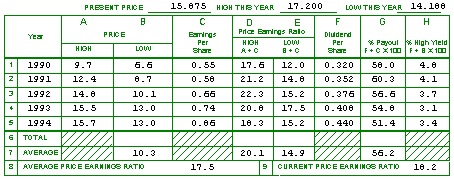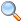Clubs Webinars Investing SSG Tutorial Blog

Section 3: Price-Earnings History as an indicator of the future

### Entering the Data

For this section, you need the high and low prices, earnings per share and dividends for each of the last five years. From this data you will be making several additional calculations.

You'll also need the stock's current price, and high and low prices for the current year. (You can use the high and low prices for the last 52 weeks from the newspaper, as these numbers are not used in any calculations but are included only for your reference.)

Record the current price, high price this year and low price this year above the chart.Then start with the oldest of the five years of data in Row 1. Enter that year's high price in Column A, the Low Price in Column B, the Earnings Per Share in Column C and the Dividends in Column F. Continue with the next four years of data, ending with the most recently completed year in Row 5.

### Calculating P/E Ratios

The next step is to calculate the high and low Price/Earnings ratios for each year. Follow the instructions in the header of the chart: for the high P/E ratio (in Column D), divide the high price (in Column A) by the EPS (in Column C). For the low P/E ratio (in Column E), divide the low price (in Column B) by the EPS (in Column C).

### Calculating Payout & High Yield Percentages

Again, follow the formulas in the chart header to determine the % Payout and % High Yield for each year. Divide the Dividends Per Share (Column F) by the Earnings Per Share (Column C) and multiply by 100 to calculate the & Payout in Column g. Divide the Dividends Per Share (Column F) by the Low Price (Column B) and record the results as the % High Yield (Column H).

### Calculating Averages

Now you need to figure out some five-year averages in Row 7. (Row 6, labelled "Total," is on the chart only for your convenience, so you don't actually need to fill it in as you calculate the averages.) Start with the Low Prices in Column B: add them up and divide by 5 to reach the Average Low Price. Record this number on the chart.

Calculate the five-year averages in the same way for Columns D and E, the High and Low P/E Ratios, and record them in Row 7. Finally, figure out the average % Payout in Column G.

Next, calculate the Average Price/Earnings Ratio in Row 8, by adding the Average High P/E Ratio and the Average Low P/E Ratio from Row 7 and dividing by 2.

For the Current Price/Earnings Ratio, record the figure from the newspaper's stock tables, or calculate it yourself by dividing the current price by the sum of the Earnings Per Share from the four most recent quarters.

### Interpreting the Results

So, what does it all mean?

Because the SSG is designed to help an investor make a reasonable projection using historical data, the figures from the past five years in this chart can be very helpful in evaluating a company's stock.

First, look at the high and low prices for the past five years (in Columns A and B). Ideally, you'd want to see both trending higher from year to year (also taking into consideration the most recent year's high and low prices on top of the chart). If the stock's price hasn't increased very much over the past five years, you may have reason to doubt that it will increase at a greater rate over the next five years.

The trading range within a year can also give you an idea about a company's price volatility. If the span between the high and low prices is consistent from year to year, or if the difference between the high and low price is similar every year, then this may provide a clue about the likelihood that the stock will trade in a similar range in the future.

Though these measures are not critical to the SSG analysis, it's a good idea to review the price data and be aware of how the stock has performed in the most recent five years.

You've already seen a graphic representation of the company's earnings per share on Page 1 of the SSG, but it can be handy to see the actual numbers here in Column C as well. In a company that doesn't pay dividends, you should be looking for a doubling of earnings per share over the next five years, in order to ensure a 15% return on your investment. So wouldn't it make sense for that company to have doubled earnings in the last five years too?

Of course, earnings aren't the whole story when you're considering the purchase of a stock--there may be possibilities of P/E enhancement (if the P/E at the time of purchase is lower than average, and subsequently returns to an average level, the price will reflect that change) and the impact of dividends. But a simple doubling of the EPS from five years ago compared to the most recent year is a quick and easily quantified double-check of how wide from your mark the company has been.

As with the high and low prices, the high and low P/E Ratios should be reviewed to see that no downward trends exist. It may be helpful to think of the stock's P/E Ratios as a proxy for its price, year by year. As the company's earnings grow, so too should its price, and if both did so evenly, the P/E Ratios would be exactly the same from year to year. However, other factors like investor sentiment and the laws of supply and demand will cause the price to fluctuate. The P/E Ratios of the past will tell you how stable the company's stock price has been in comparison to its earnings. If the P/E Ratios have been decreasing, that may be a danger signal. If the P/E Ratios have increased somewhat or remained the same, there's probably not much to worry about.

Columns D and E provide the raw data for one of the most important assessments of a company's value that isn't on the SSG! It is Relative Value, the comparison of a stock's current P/E ratio to its average P/E ratio. This figure is usually displayed as a percentage, and ideally is less than 100% (meaning that the stock's P/E ratio is lower than its average). To calculate Relative Value, divide Field 9, Current P/E Ratio, by Field 8, Average P/E Ratio.

You can compare the Current P/E ratio to the P/E ratios over the last five years--if the current P/E is at the low end of the range, the stock may be undervalued or fairly valued (assuming of course that its growth potential is strong). If the P/E ratio is at the high end of its five year history, that may be a signal that the company is temporarily overvalued. If the company's current P/E ratio is even lower than its average P/E ratio, it may be a good sign for a bargain shoppers that a sale is in progress!

The dividend and payout section is not usually much of a concern for investors looking for stocks with the maximum potential for capital appreciation. Still, dividends can provide the extra "kicker" to allow the stock's total return to exceed 15% over time.

You should consider if any trend in the dividend or payout ratio exists. If the company has been growing its earnings over the past five years, then the dividend should also be growing. If the payout ratio is very high, the company may not have enough capital to invest in itself.

### Next